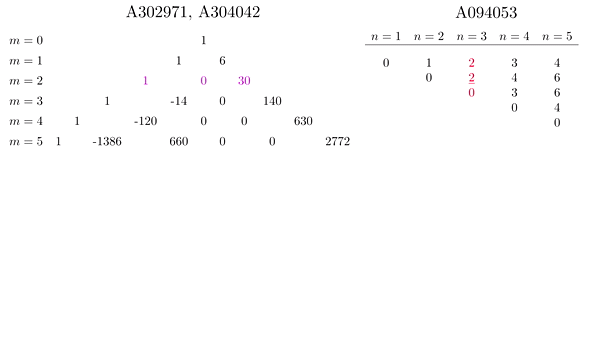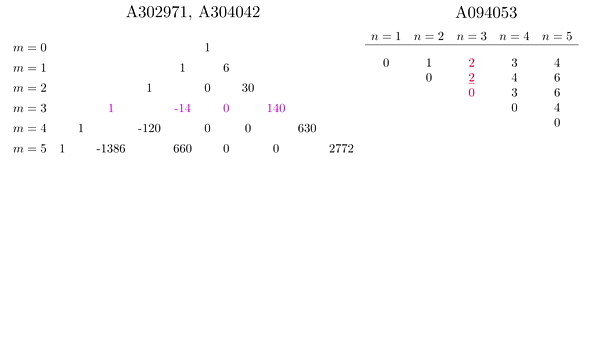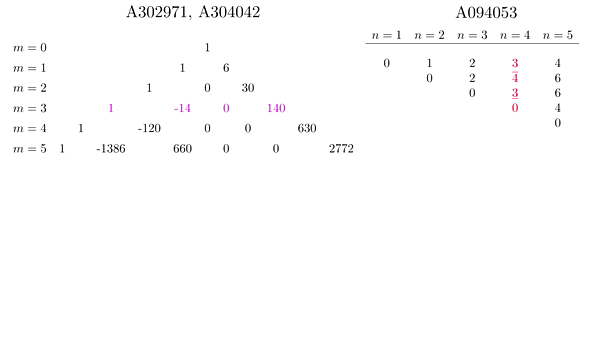# Petro Kolosov

Mathematics is the language, where lie not exist

kolosovp94@gmail.com## About Me

Very interested in Computer Science, in particular, in .NET enviroment. During my free time I do Math, my interests in math are about Faulhaber's formula, Binomial theorem and other realted to power identities topics.## Publications

• ### An odd-power identity involving discrete convolution

Let be a power function $$f_{r,M}(s)$$ defined for every $$s$$ within the finite set $$M$$ as follows $$f_{r,M}(s)= \begin{cases} s^r, \ &s\in M,\\ 0, \ &\mathrm{otherwise}. \end{cases}$$ Let a discrete convolution of $$f_{r,M}(s)$$ be denoted as follows $$\mathrm{Conv}_{r,M}[n]=(f_{r,M}*f_{r,M})[n]$$. Let a real coefficients $$A_{m,j}$$ be given by the following recurrence \begin{equation*} A_{m,j}= \begin{cases} 0, & \mathrm{if } \ j<0 \ \mathrm{or } \ j>m, \\ (2j+1)\binom{2j}{j} \sum_{d=2j+1}^{m} A_{m,d} \binom{d}{2j+1} \frac{(-1)^{d-1}}{d-j} B_{2d-2j}, & \mathrm{if } \ 0 \leq j < m, \\ (2j+1)\binom{2j}{j}, & \mathrm{if } \ j=m. \end{cases} \end{equation*} In this paper we show that for every $$n>0$$ the following odd-power identities involving coefficients $$A_{m,j}$$ and convolution transform $$\mathrm{Conv}_{r,M}[n]$$ hold \begin{equation*} \begin{split} n^{2m+1}+1&=\sum_{r=0}^{m}A_{m,r}\mathrm{Conv}_{r,\mathbb{N}}[n],\\ n^{2m+1}-1&=\sum_{r=0}^{m}A_{m,r}\mathrm{Conv}_{r,\mathbb{Z}_{>0}}[n],\\ n^{2m+1}&=\sum_{r=0}^{m}A_{m,r}\sum_{k=1}^{n} k^r(n-k)^r\\ &=\sum_{r=0}^{m}A_{m,r}\sum_{k=0}^{n-1} k^r(n-k)^r. \end{split} \end{equation*}

• Related OEIS sequences: Numerators and Denominators of $$A_{m,r}$$: A302971, A304042
• MSC classes: 11C08, 44A35
• Comments: 5 pages
• Keywords: Power Identities, Polynomials, Convolution, Convolution power, Integral transforms
• Old version: old_version

• ### On the link between finite differences and derivatives of polynomials

The main aim of this paper to establish the relations between forward, backward and central finite difference and divided difference (that is discrete analog of the derivative) and partial and ordinary high-order derivatives of the polynomials.

• MSC classes: 46G05, 30G25, 39-XX
• Comments: 12 pages, 2 figures
• Keywords: Finite difference, Divided difference, Derivative, Polynomials

• ### On the quantum differentiation of smooth real-valued functions

Calculating the value of $$C^{k\in\{1,\infty\}}$$ class of smoothness real-valued function's derivative in point of $$\mathbb{R}^+$$ in radius of convergence of its aylor polynomial (or series), applying an analog of Newton's binomial theorem and $$q$$-difference operator. $$(P,q)$$-power difference introduced in section 5. Additionally, by means of Newton's interpolation formula, the discrete analog of Taylor series interpolation using $$q$$-difference and $$p,q$$-power difference is shown.

• MSC classes: 26A24, 05A30, 41A58
• Comments: 12 pages, 6 figures
• Keywords: Quantum algebra, Differential calculus, Taylor's formula, Approximations

• ### Some notes and short reviews## Contributions to the OEIS

OIES stands for the On-Line Encyclopedia of Integer Sequences, established by Neil J. Sloane in 1965 and since 1996 is published online. Here are some sequences contributed by me.## Support my research

Dear readers! If you found my research interesting and progressive, you are free to help me by donations. Since mathematics is not my main affiliation, I don't receive any money for my work - I work for idea. I never care about money, but I have to live :) Each collected donation will be spend only to proceed my research without delays.
• For more info and details concerning donation, proceed by the link## Interesting links## GIFs and Videos

• ### Example of use of the identity reached at Another Power Identity involving Binomial Theorem and Faulhaber's formula

Consider the identity (arXiv:1603.02468v15, p. 1.), that is $$(\star)\quad\quad P_m(\ell,T) := \sum_{k=1}^{\ell}\sum_{j=0}^m A_{m,j}k^j(T-k)^j=T^{2m+1},$$ as $$\ell=T$$. To show an example of use of above identity, consider the triangle of $$A_{m,j}$$ coefficients, the sequences A302971, A304042 for the numerators and denominators, respectively. Recall the values of $$k(n-k), \ 1\leq k \leq n$$, the sequence A094053. Consider the case $$T=n=3$$ and $$m=2$$ in $$(\star)$$. So, we keep our attention to the second row of triangle A302971/A304042 and to the third row of the the sequence $$k(n-k), \ 1\leq k \leq n$$, A094053. The following GIF shows exactly the process.For the case $$T=n=3, \ m=3$$ in $$(\star)$$, we have, respectivelyAnd for the case $$T=n=4, \ m=3$$ in $$(\star)$$, we haveAll of the above examples are aveilable on youtube as follows## Mathematica codes

Here is some important Mathematica codes, in general, concerning to the preprint arXiv:1603.02468.

• Code 1 - displays the triangular array of coefficients $$A_{m,j}$$, see arXiv:1603.02468v15, p. 12, table 4.

• Code 2 - verifies the identity $$P_{m}(\ell,T)=\sum\limits_{1\leq k \leq\ell}\sum\limits_{0\leq j\leq m} A_{m,j}k^j(T-k)^j=T^{2m+1}$$ as $$\ell=T$$, see arXiv:1603.02468v15, p. 1, equation (1.1).

• Code 3 - verifies the identity $$P_{m}(\ell,T)=\sum\limits_{0\leq k\leq m}(-1)^{m-k}U_{m}(\ell, k)\cdot T^k=T^{2m+1}$$ as $$\ell=T$$, see arXiv:1603.02468v15, p. 1, equation (1.1).

• Code 4 - prints the tabular consisting the coefficients $$U_m(\ell,k)$$, for particular $$m\in\mathbb{N}$$. By default $$m=2$$.

• Code 5 - produces the list of polyomials $$P_{m}(\ell,T)=\sum\limits_{0\leq k\leq m}(-1)^{m-k}U_{m}(\ell, k)\cdot T^k$$ for particular $$m\in\mathbb{N}$$ and $$1\leq \ell \leq 40$$, by default $$m=2$$.

• Code 6 - prints the tabular of the coefficients $$\mathcal{V}_{m}(\ell,k)$$, defined by the identity $$\sum\limits_{0\leq k \leq\ell-1}\sum\limits_{0\leq j\leq m} A_{m,j}k^j(T-k)^j=\sum\limits_{0\leq k\leq m}(-1)^{m-k}\mathcal{V}_{m}(\ell,k)\cdot T^k=T^{2m+1}$$ as $$\ell=T$$, see arXiv:1603.02468v12, pp. 19-20, expression (4.17), (4.18), figure (4.19).

• Code 7 - verifies the identity $$\sum\limits_{0\leq k\leq m}(-1)^{m-k}\mathcal{V}_{m}(\ell,k)\cdot T^k=T^{2m+1}$$ as $$\ell=T$$, see arXiv:1603.02468v12, p. 19, expression (4.18).

• Code 8 - produces the list of polynomials $$\sum\limits_{0\leq k\leq m}(-1)^{m-k}\mathcal{V}_{m}(\ell,k)\cdot T^k=T^{2m+1}$$ as $$\ell=T$$ for $$\ell=1, ..., 40$$, see arXiv:1603.02468v12, p. 20, figure (4.19).

• Code 9 - produces the list of the "combined" coefficients $$\mathcal{U}_m(\ell,k)$$, defined by $$\mathcal{U}_m(\ell,k)=\frac{1}{2}[U_m(\ell,k)+\mathcal{V}_{m}(\ell,k)]$$, such that $$\sum\limits_{0\leq k\leq m}(-1)^{m-k}\mathcal{U}_{m}(\ell,k)\cdot T^k=T^{2m+1}$$ as $$\ell=T$$, see arXiv:1603.02468v12, p. 21, expression (4.22).

• Code 10 - produces the list of polynomials $$\sum\limits_{0\leq k\leq m}(-1)^{m-k}\mathcal{U}_{m}(\ell,k)\cdot T^k=T^{2m+1}$$ as $$\ell=T$$ for $$\ell=1, ..., 40$$, see arXiv:1603.02468v12, p. 21, figure (4.23).

• Code 11 - verifies the identity $$\sum\limits_{0\leq k\leq m}(-1)^{m-k}\mathcal{U}_{m}(\ell,k)\cdot T^k=T^{2m+1}$$ as $$\ell=T$$, see arXiv:1603.02468v12, p. 21, expression (4.22).